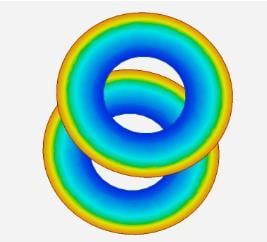## AIM Tutorials

•svecchi
Ansys EmployeeA viscous fluid is between two concentric cylinders of radii a and b, which are rotating at constant angular velocities. The diagram below shows these two cylinders and their respective angular velocities. In this problem, a=1[m] b=2a and 2=0 [rad/s] but the velocity of the inner wall must be calculated to create the Taylor-Couette phenomenon. Find the velocity vectors that are characteristic of the Taylor-Couette flow. See the attached file for the tutorial regarding this problem.

•swathi
Subscriber

Can you suggest me a better turbulent model that can be used? I find that only RSM model can be used. But I didn't find many tutorials for it.

•praveen98
Subscriber

Madam, me too working on similar problem. Can u share any material if you had found any